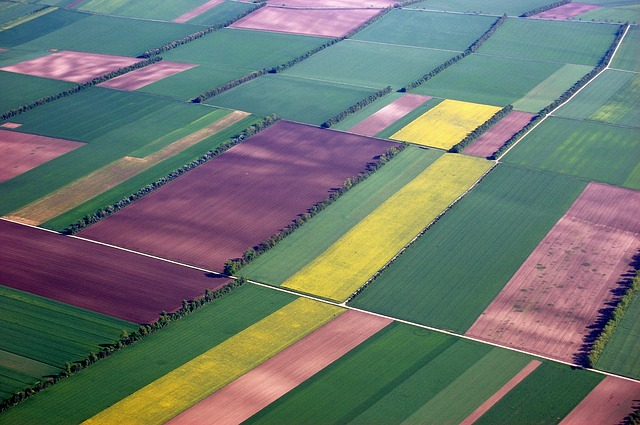# Basic & Composite Figures

Student View (Opens in new window)# Lesson Overview

Students use what they know about finding the areas of basic figures to find areas of composite figures.

# Key Concepts

A composite figure is a figure that can be divided into two or more basic figures.

The area of a composite figure can be found by dividing it into basic figures whose areas can be calculated easily.

For some figures, the area can also be found by surrounding the figure with a basic figure, creating other basic figures “between” the original figure and the surrounding figure. The area of the original figure can then be found by subtracting the basic figure.

# Goals and Learning Objectives

• Find the area of composite figures by decomposing and composing them into more basic figures.